## A cable with a linear density of \mu=0.109~\text{kg/m}μ=0.109 kg/m is hung from telephone poles. The tension in the cable is 572 N. The dist

Question

A cable with a linear density of \mu=0.109~\text{kg/m}μ=0.109 kg/m is hung from telephone poles. The tension in the cable is 572 N. The distance between poles is 19.9 meters. The wind blows across the line, causing the cable resonate. A standing waves pattern is produced that has 4.5 wavelengths between the two poles. Assuming room temperature air, what is the frequency of the hum?

in progress 0
6 months 2021-07-30T00:18:47+00:00 1 Answers 7 views 0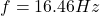Explanation:

The equation of the speed of a mechanical wave in terms of the tension and linear density, of the cable in our case, is given by: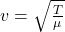Where:

• T is the tension of the cable (T = 572 N)
• μ is the linear density of the cable (μ = 0.109 kg/m)

And we know that v = λ*f

• λ is the wavelength
• f is the frequency

Because a standing waves pattern is produced that has 4.5 wavelengths between the two poles and the distance between poles is 19.9 meters, the value of the wavelength is: λ = 19.9/4.5 = 4.4 m.

Therefore, the frequency will be: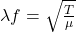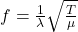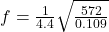I hope it helps you!